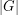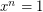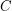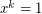# Subgroup formed by elements of order dividing n

 Importance: Medium ✭✭
 Author(s): Frobenius, Ferdinand G.
 Subject: Group Theory
 Keywords: order, dividing
 Posted by: dlh12 on: January 28th, 2008
Conjecture

Supposeis a finite group, andis a positive integer dividing. Suppose thathas exactlysolutions to. Does it follow that these solutions form a subgroup of?

If these solutions form a subgroup, they form a characteristic (and therefore normal) subgroup of. This easily follows from the First Sylow Theorem ifis the highest power of a primedividing.

In a 1980 article, Feit commented that the case where(i.e.,'exactly divides') had been reduced to consideringsimple. Thus it should be resolvable using the classification of finite simple groups.

It is known that ifdivides, the number of solutions ofinis a multiple of. A generalization of this theorem, replacingbyfor a conjugacy classof, can be found in Marshall Hall Jr.'s book.

Observation   This conjecture implies the (known) theorem of Frobenius:

Theorem   Ifis a finite transitive permutation group in which only the identity has more than one fixed point, then the derangements of, together with the identity, form a subgroup of.

To prove Frobenius' Theorem from this (sayis such a permutation group onpoints), use the standard elementary counting arguments to show that the solutions toare only the derangements and the identity and that there are exactlyof these. This theorem of Frobenius has not yet been proven in general without the use of group characters.

## Bibliography

Marshall Hall Jr., Theory of Groups, Macmillan (1959)

Walter Feit, On a Conjecture of Frobenius, Proceedings of the American Mathematical Society, Vol.7, No. 2 (Apr. 1956), 177-187.

* indicates original appearance(s) of problem.

### Solved... again

it can be proved by a isomorphism between the solutions of x^n=1 in G and the solutions of the same eq in C (complex numbers).

### Solved

This conjecture has been proven.